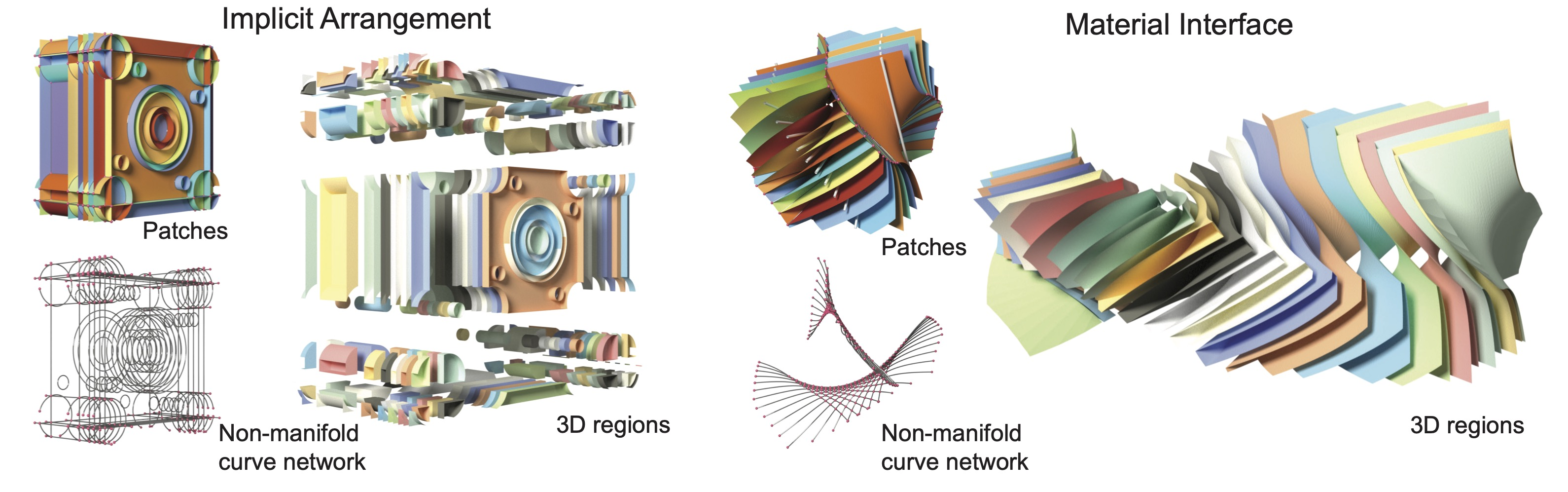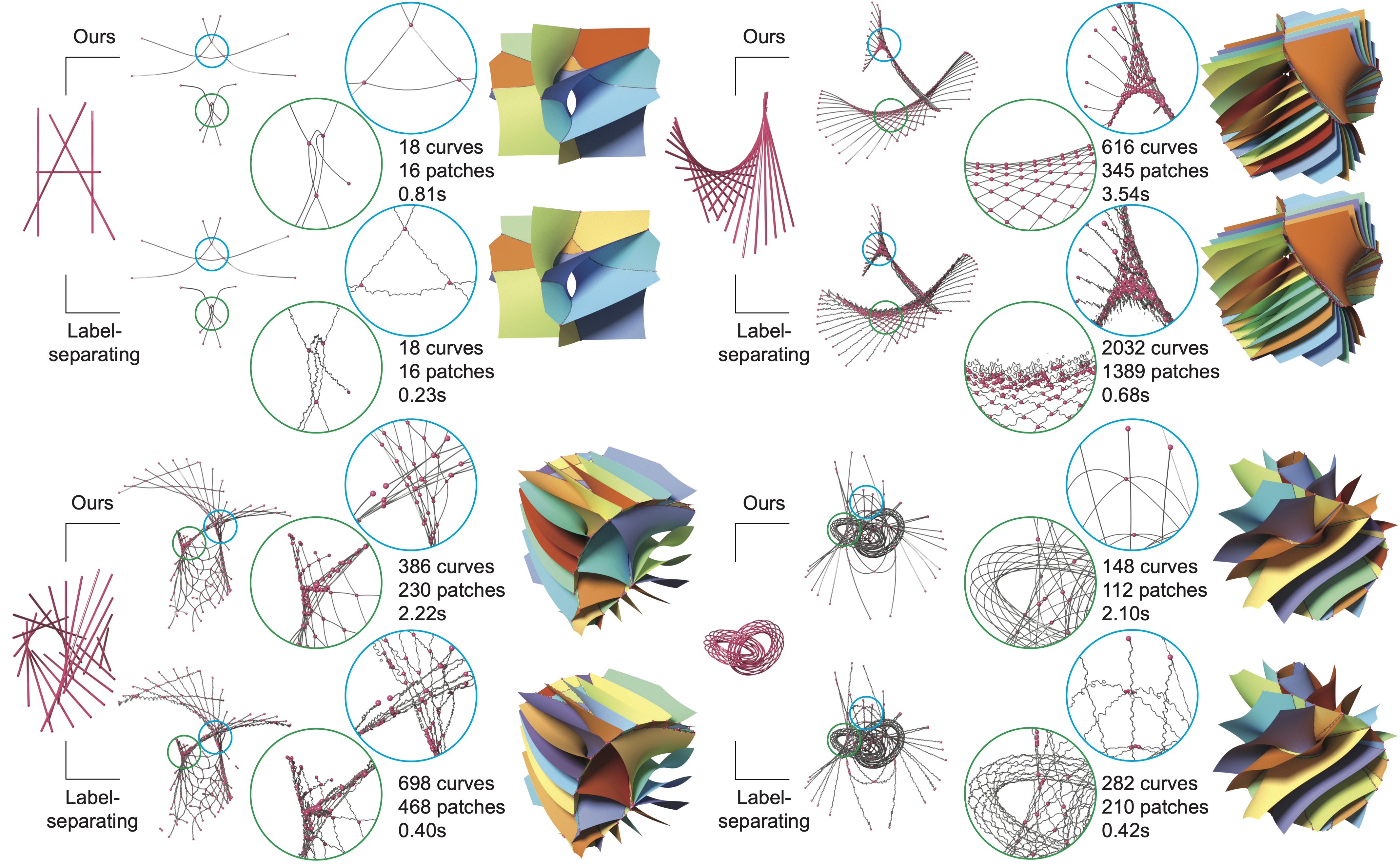# Robust Computation of Implicit Surface Networks for Piecewise Linear FunctionsFigure 1. Implicit surface networks, such as implicit arrangement (left, obtained for primitive geometry defining a CAD object) and material interfaces (right, the Voronoi diagram of rotating 3D lines), produced by our robust algorithms. Each example is visualized by its surface patches, non-manifold curve network as well as the 3D regions partitioned by the surface network.

### Abstract

Implicit surface networks, such as arrangements of implicit surfaces and materials interfaces, are used for modeling piecewise smooth or partitioned shapes. However, accurate and numerically robust algorithms for discretizing either structure on a grid are still lacking. We present a unified approach for computing both types of surface networks for piecewise linear functions defined on a tetrahedral grid. Both algorithms are guaranteed to produce a correct combinatorial structure for any number of functions. Our main contribution is an exact and efficient method for partitioning a tetrahedron using the level sets of linear functions defined by barycentric interpolation. To further improve performance, we designed look-up tables to speed up processing of tetrahedra involving few functions and introduced an efficient algorithm for identifying nested 3D regions.

## ResultsFigure 2. Implict arrangements computed by our algorithm on implicit functions representing CAD models. Each example shows the non-manifold curve networks, patches, and 3D regions (in exploded view). Complexity of each example, running time of our method (full pipeline) and mesh arrangement are noted.Figure 3. Voronoi diagrams of 3D lines (top left: 5 rotating lines; top right: 20 rotating lines; bottom left: 21 lines that sweep a Mobius strip) and circles (bottom right: 22 Villarceau circles on two tori) computed by our algorithm and the label-separating algorithm. The zoom-in views highlight regions on the non-manifold curve networks where the two algorithms produce notably different geometry and/or topology. The combinatorial complexity of each surface network and the running times (up to mesh extraction, before space decomposition) are noted.